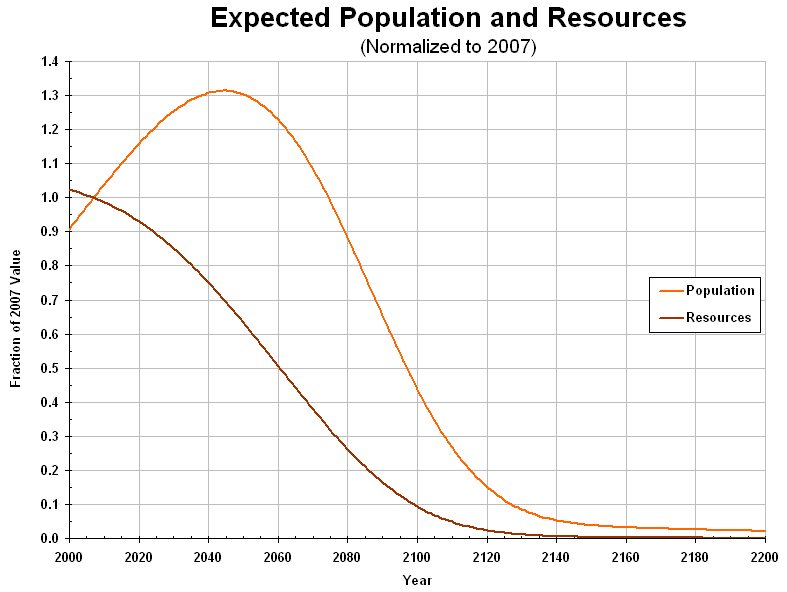Consumption Model

The consumption model is based on the observation that world population is highly correlated with cumulative consumption (the total amount of resources consumed since a given point in time) for ecological resources and energy.

Starting with an amount of some kind of resource, a group of people (the "population") begins to consume it. Some of the resources are reusable or renewable, so they can be consumed without depleting the total amount of resources (beyond this "capacity" the consumed resources are removed from the total).

Resources may otherwise exponentially increase or decrease. The capacity can also exponentially increase or decrease.

If the amount of resources decreases, then the size of the population (after its natural growth) decreases proportionately to the amount of resources due to a desire to maintain the amount of per capita consumption. If the amount of resources increases, the population experiences only natural growth.

Where the resources involved are used by the entire world, the "population" is the world population.

BELOW: World population and resources, as fractions of their calculated values at the end of 2007. This graph combines projections from consumption of ecological resources (measured by the Global Ecological Footprint), energy (production), and economic resources (measured by the Gross World Product).Consumption of three types of resources are simulated: ecological ("footprint"), energy, and economic ("GWP"). Several representative scenarios are described on this site: no change ("business as usual"), no growth (of population and per capita consumption), and high growth (increasing resources to maintain current trends in the growth of population and per capital consumption).

See the following: